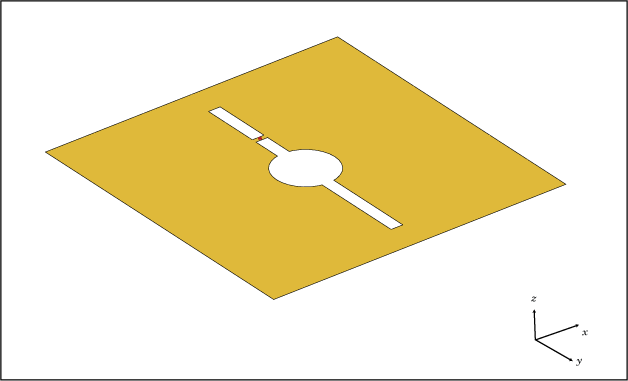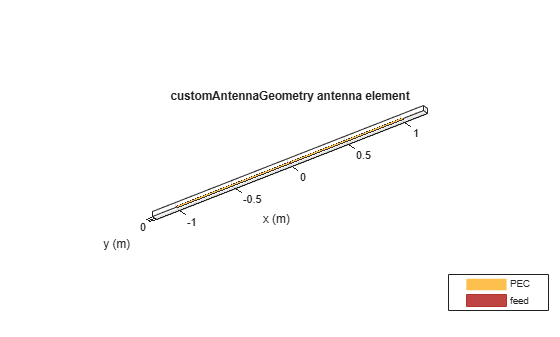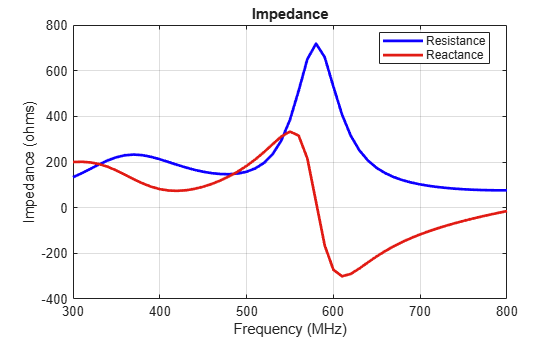# customAntennaGeometry

Create antenna represented by 2-D custom geometry

## Description

The `customAntennaGeometry` object is an antenna represented by a 2-D custom geometry on the X-Y plane. Using `customAntennaGeometry`, you can import a planar mesh, define the feed for this mesh to create an antenna, analyze the antenna, and use it in finite or infinite arrays. The image shown is a custom slot antenna.## Creation

### Syntax

``ca = customAntennaGeometry``
``ca = customAntennaGeometry(Name,Value)``

### Description

example

``` `ca = customAntennaGeometry` creates a 2-D antenna represented by a custom geometry, based on the specified boundary.```

example

``` `ca = customAntennaGeometry(Name,Value)` creates a 2-D planar antenna geometry, with additional properties specified by one or more name-value pair arguments. `Name` is the property name and `Value` is the corresponding value. You can specify several name-value pair arguments in any order as `Name1`, `Value1`, `...`, `NameN`, `ValueN`. Properties not specified retain their default values.```

## Properties

expand all

Boundary information in Cartesian coordinates, specified as a cell array in meters.

Data Types: `double`

Boolean operation performed on the boundary list, specified as a character vector.

Example: `'Operation','P1-P2'`

Data Types: `double`

Antenna feed location in Cartesian coordinates, specified as a three-element vector. The three-element vector is the X, Y, and Z coordinates respectively.

Example: `'FeedLocation', [0 0.2 0]`

Data Types: `double`

Width of feed section, specified as a scalar in meters.

Example: `'FeedWidth',0.05`

Data Types: `double`

Lumped elements added to the antenna feed, specified a lumped element object handle. For more information, see `lumpedElement`.

Example: `'Load'`, lumpedelement. `lumpedelement` is the object handle for the load created using `lumpedElement`.

Tilt angle of antenna, specified as a scalar or vector with each element unit in degrees.

Example: `'Tilt',90`

Example: `'Tilt',[90 90 0]`

Data Types: `double`

Tilt angle of the antenna, specified as a scalar or vector with each element unit in degrees. For more information, see Rotate Antennas and Arrays.

Example: `'Tilt',90`

Example: `'Tilt',[90 90]`,`'TiltAxis',[0 1 0;0 1 1]` tilts the antenna at 90 degree about two axes, defined by vectors.

Data Types: `double`

Tilt axis of the antenna, specified as:

• Three-element vectors of Cartesian coordinates in meters. In this case, each vector starts at the origin and lies along the specified points on the X-, Y-, and Z-axes.

• Two points in space, each specified as three-element vectors of Cartesian coordinates. In this case, the antenna rotates around the line joining the two points in space.

• A string input describing simple rotations around one of the principal axes, 'X', 'Y', or 'Z'.

Example: `'TiltAxis',[0 1 0]`

Example: `'TiltAxis',[0 0 0;0 1 0]`

Example: `ant.TiltAxis = 'Z'`

## Object Functions

 `show` Display antenna or array structure; Display shape as filled patch `info` Display information about antenna or array `axialRatio` Axial ratio of antenna `beamwidth` Beamwidth of antenna `charge` Charge distribution on metal or dielectric antenna or array surface `current` Current distribution on metal or dielectric antenna or array surface `design` Design prototype antenna or arrays for resonance at specified frequency `EHfields` Electric and magnetic fields of antennas; Embedded electric and magnetic fields of antenna element in arrays `impedance` Input impedance of antenna; scan impedance of array `mesh` Mesh properties of metal or dielectric antenna or array structure `meshconfig` Change mesh mode of antenna structure `pattern` Radiation pattern and phase of antenna or array; Embedded pattern of antenna element in array `patternAzimuth` Azimuth pattern of antenna or array `patternElevation` Elevation pattern of antenna or array `returnLoss` Return loss of antenna; scan return loss of array `sparameters` S-parameter object `show` Display antenna or array structure; Display shape as filled patch `vswr` Voltage standing wave ratio of antenna

## Examples

collapse all

Create a custom dipole antenna and view it.

`ca = customAntennaGeometry`
```ca = customAntennaGeometry with properties: Boundary: {[4x3 double]} Operation: 'P1' FeedLocation: [0 0 0] FeedWidth: 0.0200 Tilt: 0 TiltAxis: [1 0 0] Load: [1x1 lumpedElement] ```
`show(ca)`Create a custom slot antenna using three rectangles and a circle.

Make three rectangles of 0.5 m x 0.5 m, 0.02 m x 0.4 m and 0.03 m x 0.008 m.

```pr = em.internal.makerectangle(0.5,0.5); pr1 = em.internal.makerectangle(0.02,0.4); pr2 = em.internal.makerectangle(0.03,0.008); ```

Make a circle of radius 0.05 m.

```ph = em.internal.makecircle(0.05); ```

Translate the third rectangle to the X-Y plane using the coordinates [0 0.1 0].

```pf = em.internal.translateshape(pr2,[0 0.1 0]); ```

Create a custom slot antenna element using the specified boundary shapes. Transpose pr, ph, pr1, and pf to make sure the boundary inputs are column vector arrays.

```c = customAntennaGeometry('Boundary',{pr',ph',pr1',pf'},... 'Operation','P1-P2-P3+P4'); figure; show(c); ```Move the feed location to new coordinates.

```c.FeedLocation = [0,0.1,0]; figure; show(c); ```Analyze the impedance of the antenna from 300 MHz to 800 MHz.

```figure; impedance(c, linspace(300e6,800e6,51)); ```Analyze the current distribution of the antenna at 575 MHz.

```figure; current(c,575e6) ```Plot the radiation pattern of the antenna at 575 MHz.

```figure; pattern(c,575e6) ```Balanis, C. A. Antenna Theory. Analysis and Design. 3rd Ed. Hoboken, NJ: John Wiley & Sons, 2005.